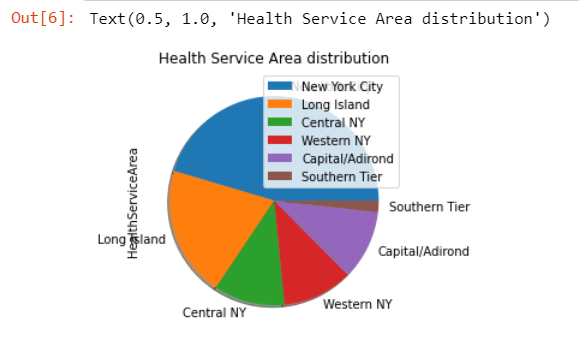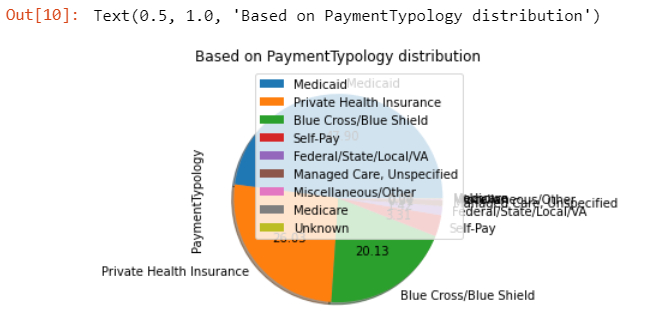Search

# Predict Length Of Stay (LOS) Of a Patient Recent COVID-19 Pandemic### Introduction

Hospitals are constantly challenged to provide timely patient care while maintaining high resource utilization. While this challenge has been around for many years, the recent COVID-19 pandemic has increased its prominence. For a hospitals, the ability to predict length of stay (LOS) of a patient as early as possible (at the admission stage) is very useful in managing its resources.

In this task, you will develop a ML model to predict if a patient will be discharged from a hospital early or, will stay in hospital for an extended period (see task below for exact definition), based on several attributes (features) related to: patient characteristics, diagnoses, treatments, services, hospital charges and patients socio-economic background.

The machine learning task we are interested in is: “Predict if a given patient (i.e. newborn child) will be discharged from the hospital within 3 days (class 0) or will stay in hospital beyond that - 4 days or more (class 1)”.

The data set to develop your models is given to you on canvas. Note that you need to transform the target column (“LengthOfStay”) to match the two classes mentioned in the above task. Class 0 if LengthOfStay < 4 and class 1 otherwise.

• You need to come up with an approach (that follows the restrictions in 3.2), where each element of the system is justified using data analysis, performance analysis and/or knowledge from relevant literature.

• As one of the aims of the assignment is to become familiar with the machine learning paradigm, you should evaluate multiple different models (only use techniques taught in class up to week 5 - inclusive) to determine which one is most appropriate for this task.

• Setup an evaluation framework, including selecting appropriate performance measures, and determining how to split the data.

• Finally you need to analyse the model and the results from your model using appropriate techniques and establish how adequate your model is to perform the task in real world and discuss limitation if there are any (ultimate judgement).

• Predict the result for the test set.

### Dataset

The data set for this assignment is available on Canvas. There are the following files:

• “train data.csv”: Contain the train set, attributes and target for each patient. This data is to be used in developing the models. Use this for your own exploration and evaluation of which approach you think is “best” for this prediction task.

• “test data.csv”: Contain the test set, attributes for each patient. You need to make predictions for this data and submit the prediction via canvas. The teaching team will use this data to evaluate the performance of the model you have developed.

• “s1234567 predictions.csv”: Shows the expected format for your predictions on the unseen test data. You should organize your predictions in this format. Any deviation from this format will result on zero marks for the results part. Change the number in filename to your student ID.

### Implementation

Import Libraries

```# Convolutional Neural Network
# Importing the libraries
import tensorflow as tf
import pandas as pd
import numpy as np
from tensorflow.keras.preprocessing.image import ImageDataGenerator
import matplotlib.pyplot as plt
from tensorflow.keras.layers import Conv2D,Dropout
from sklearn.preprocessing import LabelEncoder
from xgboost import XGBClassifier
from matplotlib import pyplot
from sklearn.linear_model import LogisticRegression
from sklearn.preprocessing import OneHotEncoder
from sklearn.model_selection import train_test_split,GridSearchCV,cross_val_score
from sklearn.metrics import classification_report
from sklearn.metrics import confusion_matrix
from sklearn.svm import SVC```

```train_df=pd.read_csv('train_data.csv')
train_df```

Output ResultDescribe the Dataset

`display(train_df.describe())`

Output:Check if any null vaule present or not

```#check for missing values
train_df.isnull().values.any()```

Output

`False`

### Data Visualization

```# Class wise(Normal & Fraud) distribution of data
count_classes = pd.value_counts(train_df['HealthServiceArea'], sort = True)
plt.title("Health Service Area distribution")

```

Output:```
# Class Gender distribution of data
count_classes = pd.value_counts(train_df['Gender'], sort = True)
plt.title("Gender distribution")```

Output:```# Class wise(Normal & Fraud) distribution of data
count_classes = pd.value_counts(train_df['Race'], sort = True)
plt.title("Based on Race distribution")```

Output:```# Class wise(Normal & Fraud) distribution of data
count_classes = pd.value_counts(train_df['TypeOfAdmission'], sort = True)

Output:```# Class wise(Normal & Fraud) distribution of data
count_classes = pd.value_counts(train_df['PaymentTypology'], sort = True)
plt.title("Based on PaymentTypology distribution")```

Output:Histograms

```plt.hist(train_df.AverageCostInFacility, label='Cost In Facility')
plt.legend(loc='upper right')
plt.xlabel('Average Cost In Facility of Transaction')
plt.ylabel('Number of Transactions')
plt.show()```

Output:### Data preprocessing

Convert length of stay to 0 and 1

`train_df['LengthOfStay'] = train_df['LengthOfStay'].apply(lambda x: 1 if x > 3 else 0)`
`print(train_df.info())`

Output:

```<class 'pandas.core.frame.DataFrame'>
RangeIndex: 59966 entries, 0 to 59965
Data columns (total 16 columns):
#   Column                        Non-Null Count  Dtype
---  ------                        --------------  -----
0   ID                            59966 non-null  int64
1   HealthServiceArea             59966 non-null  object
2   Gender                        59966 non-null  object
3   Race                          59966 non-null  object
5   CCSProcedureCode              59966 non-null  int64
6   APRSeverityOfIllnessCode      59966 non-null  int64
7   PaymentTypology               59966 non-null  object
8   BirthWeight                   59966 non-null  int64
9   EmergencyDepartmentIndicator  59966 non-null  object
10  AverageCostInCounty           59966 non-null  int64
11  AverageChargesInCounty        59966 non-null  int64
12  AverageCostInFacility         59966 non-null  int64
13  AverageChargesInFacility      59966 non-null  int64
14  AverageIncomeInZipCode        59966 non-null  int64
15  LengthOfStay                  59966 non-null  int64
dtypes: int64(10), object(6)
memory usage: 7.3+ MB
None```

Remove Id and HealthServiceArea as per requirement

```remove_col=[]
cat_col=[]```
```train_df.drop(['ID', 'HealthServiceArea'], axis=1, inplace=True)
remove_col.append('ID')
remove_col.append('HealthServiceArea')```

Transform Gender with label encoder to convert categorical data to numerical

```# creating instance of labelencoder
Gender_labelencoder = LabelEncoder()

# Assigning numerical values and storing in another column
train_df['Gender'] = Gender_labelencoder.fit_transform(train_df['Gender'])
cat_col.append('Gender')```

Convert categorical data to numerical

Transform Race with label encoder

```# creating instance of labelencoder
Race_labelencoder = LabelEncoder()

# Assigning numerical values and storing in another column
train_df['Race'] = Race_labelencoder.fit_transform(train_df['Race'])
cat_col.append('Race')```
`train_df['TypeOfAdmission'].value_counts()`

Output:

```Newborn      58741
Emergency      659
Urgent         412
Elective       154

```# creating instance of labelencoder

# Assigning numerical values and storing in another column

```
`train_df['CCSProcedureCode'].value_counts()`

Output:

```228    19886
115    13628
0      11189
220    10773
231     2981
-1        769
216      740
Name: CCSProcedureCode, dtype: int64```

Transform CCSProcedureCode with label encoder

```# creating instance of labelencoder
CCSProcedureCode_labelencoder = LabelEncoder()

# Assigning numerical values and storing in another column
train_df['CCSProcedureCode'] = CCSProcedureCode_labelencoder.fit_transform(train_df['CCSProcedureCode'])
cat_col.append('CCSProcedureCode')```
`train_df['APRSeverityOfIllnessCode'].value_counts()`

Output:

```1    47953
2     8760
3     3252
4        1
Name: APRSeverityOfIllnessCode, dtype: int64```

`train_df['PaymentTypology'].value_counts()`

Output:

```Medicaid                     28723
Private Health Insurance     15608
Blue Cross/Blue Shield       12073
Self-Pay                      1984
Federal/State/Local/VA         849
Managed Care, Unspecified      545
Miscellaneous/Other            118
Medicare                        44
Unknown                         22
Name: PaymentTypology, dtype: int64```

Transform PaymentTypology with label encoder

```# creating instance of labelencoder
PaymentTypology_labelencoder = LabelEncoder()

# Assigning numerical values and storing in another column
train_df['PaymentTypology'] = PaymentTypology_labelencoder.fit_transform(train_df['PaymentTypology'])
cat_col.append('PaymentTypology')

```
`train_df['EmergencyDepartmentIndicator'].value_counts()`

<